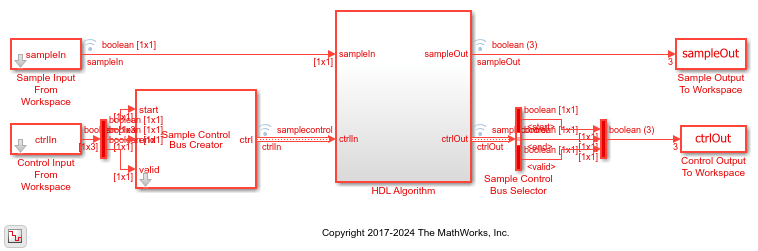whdlSamplesToFrames

Convert sample stream to frame-based data

Description

example

outframes = whdlSamplesToFrames(samples,ctrl) composes frame-based data from a sample stream and corresponding control signals. The control signals indicate the validity of the samples and the boundaries of the frames. The function calculates the maximum frame length from the input data and control signals, and removes any idle or nonvalid samples from the data.

example

outframes = whdlSamplesToFrames(samples,ctrl,maxlen) composes frame-based data, using the maximum frame length. If an input frame described by samples is larger than maxlen, the function truncates the frame.

example

outframes = whdlSamplesToFrames(samples,ctrl,maxlen,interleaved) orders the frame-based data, assuming the input samples are interleaved, when interleaved is 1 (true). The interleaved argument is valid only when each sample is represented by multiple values. The function computes the number of values representing each sample by comparing the length of samples and ctrl.

Examples

collapse all

This example shows how to use the LTE Turbo Encoder block to encode data, and how to compare the hardware-friendly design with the results from LTE Toolbox™. The workflow follows these steps:

1. Generate frames of random input samples in MATLAB®.

2. Encode the data using the LTE Toolbox function lteTurboEncode.

3. Convert framed input data to a stream of samples and import the stream into Simulink®.

4. To encode the samples using a hardware-friendly architecture, run the Simulink model, which contains the Wireless HDL Toolbox™ block LTE Turbo Encoder.

5. Export the stream of encoded samples to the MATLAB workspace.

6. Convert the sample stream back to framed data, and compare the frames with the reference data.

Generate input data frames. Generate reference encoded data using lteTurboEncode.

rng(0);
turboframesize = 40;
numframes = 2;

txBits    = cell(1,numframes);
codedData = cell(1,numframes);

for ii = 1:numframes
txBits{ii} = logical(randi([0 1],turboframesize,1));
codedData{ii} = lteTurboEncode(txBits{ii});
end

Serialize input data for the Simulink model. Leave enough time between frames for each frame to be fully encoded before the next one starts. The LTE Turbo Encoder block takes inframesize + 16 cycles to complete encoding of a frame.

inframes = txBits;

inframesize = size(inframes{1},1);

idlecyclesbetweensamples = 0;
idlecyclesbetweenframes = inframesize+16;

[sampleIn,ctrlIn] = ...
whdlFramesToSamples(inframes, ...
idlecyclesbetweensamples, ...
idlecyclesbetweenframes);

Run the Simulink model. The simulation time equals the number of input samples. Because of the added idle cycles between frames, the streaming input data includes enough cycles for the model to complete encoding of both frames.

sampletime = 1;
samplesizeIn = 1;
simTime = size(ctrlIn,1);
modelname = 'ltehdlTurboEncoderModel';
open_system(modelname);
sim(modelname);The Simulink model exports sampleOut_ts and ctrlOut_ts back to the MATLAB workspace. Deserialize the output samples, and compare the framed data to the reference encoded frames.

The output samples of the LTE Turbo Encoder block are interleaved with the parity bits.

Hardware-friendly output: S_1 P1_1 P2_1 S2 P1_2 P2_2 ... Sn P1_n P2_n

LTE Toolbox output: S_1 S_2 ... S_n P1_1 P1_2 ... P1_n P2_1 P2_2 ... P2_n

Reorder the samples using the interleave option of the whdlSamplesToFrames function. Compare the reordered output frames with the reference encoded frames.

sampleOut = sampleOut';
interleaveSamples = true;
outframes = whdlSamplesToFrames(sampleOut(:),ctrlOut,[],interleaveSamples);

fprintf('\nLTE Turbo Encoder\n');
for ii = 1:numframes
numBitsDiff = sum(outframes{ii} ~= codedData{ii});
fprintf(['  Frame %d: Behavioral and ' ...
'HDL simulation differ by %d bits\n'],ii,numBitsDiff);
end
Maximum frame size computed to be 132 samples.

LTE Turbo Encoder
Frame 1: Behavioral and HDL simulation differ by 0 bits
Frame 2: Behavioral and HDL simulation differ by 0 bits

Input Arguments

collapse all

Stream of output samples, specified as a column vector. The vector can include idle cycles between samples and between frames. Idle cycles are discarded. The frames represented by the stream can be different sizes. The vector length, N, must be an integer multiple of the length of the ctrl matrix, M. Differing lengths mean that each sample is represented by N/M values.

For example, in the LTE standard, the turbo code rate is 1/3, so each turbo-encoded sample is represented by one systematic, and two parity values: Sn, Pn1, and Pn2. In that case, the length of samples must be three times the length of ctrl.

Data Types: single | double | int8 | int16 | int32 | int64 | uint8 | uint16 | uint32 | uint64 | logical | fi

Control signals accompanying the sample stream, specified as an M-by-3 matrix. The matrix includes three control signals, start, end, and valid, for each sample in samples. Each sample can be represented by more than one value. In that case, the length of samples must be an integer multiple of M.

For example, in the LTE standard, the turbo code rate is 1/3, so each turbo-encoded sample is represented by one systematic, and two parity values: Sn, Pn1, and Pn2. In that case, the length of samples must be three times the length of ctrl.

Data Types: logical

Maximum frame length, specified as an integer. The input frames in samples can be different sizes. The output column vector reflects the size of the input frame, according to ctrl. If a frame is larger than maxlen, the function truncates the frame and returns a warning message.

Data Types: double

Order of output samples relative to input order, when more than one value represents each sample, specified as a logical scalar.

For example, 1/3 turbo-encoded samples are represented by [S1 P11 P12 S2 P21 P22]. To reorder the samples so that systematic and parity values are grouped together, set interleaved to 1 (true). The output order is then [S1 S2 P11 P21 P12 P22].

Data Types: logical

Output Arguments

collapse all

Frames of output samples, returned as a column vector or a cell array of column vectors. The size of the output column vector reflects the size of the input frame, as determined by the control signals in ctrl.

Data Types: single | double | int8 | int16 | int32 | int64 | uint8 | uint16 | uint32 | uint64 | logical | fi